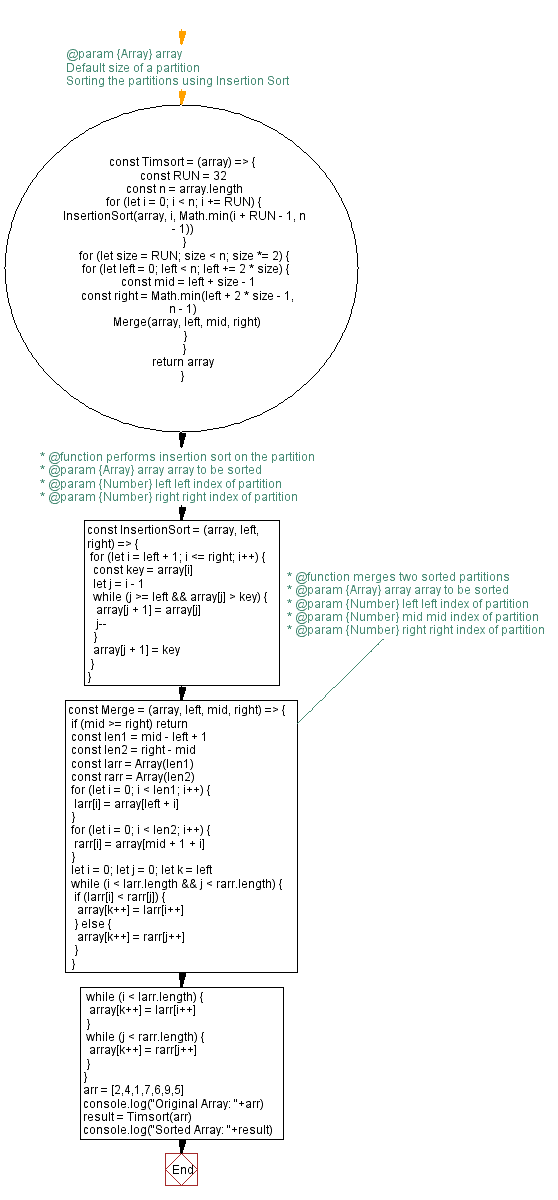# JavaScript Sorting Algorithm: Timsort sorting algorithm

## JavaScript Sorting Algorithm: Exercise-25 with Solution

From Wikipedia,
Timsort is a hybrid, stable sorting algorithm, derived from merge sort and insertion sort, designed to perform well on many kinds of real-world data. It was implemented by Tim Peters in 2002 for use in the Python programming language. The algorithm finds subsequences of the data that are already ordered (runs) and uses them to sort the remainder more efficiently. This is done by merging runs until certain criteria are fulfilled. Timsort has been Python's standard sorting algorithm since version 2.3. It is also used to sort arrays of non-primitive type in Java SE 7, on the Android platform, in GNU Octave, on V8, Swift, and Rust.

Write a JavaScript program to sort a list of elements using the Timsort sorting algorithm.

Sample Data: Original Array: 2,4,1,7,6,9,5
Sorted Array: 1,2,4,5,6,7,9

Sample Solution:

JavaScript Code:

``````//License:shorturl.at/FSX26
//  @param {Array} array

const Timsort = (array) => {
// Default size of a partition
const RUN = 32
const n = array.length
// Sorting the partitions using Insertion Sort
for (let i = 0; i < n; i += RUN) {
InsertionSort(array, i, Math.min(i + RUN - 1, n - 1))
}
for (let size = RUN; size < n; size *= 2) {
for (let left = 0; left < n; left += 2 * size) {
const mid = left + size - 1
const right = Math.min(left + 2 * size - 1, n - 1)
Merge(array, left, mid, right)
}
}
return array
}

/**
* @function performs insertion sort on the partition
* @param {Array} array array to be sorted
* @param {Number} left left index of partition
* @param {Number} right right index of partition
*/

const InsertionSort = (array, left, right) => {
for (let i = left + 1; i <= right; i++) {
const key = array[i]
let j = i - 1
while (j >= left && array[j] > key) {
array[j + 1] = array[j]
j--
}
array[j + 1] = key
}
}

/**
* @function merges two sorted partitions
* @param {Array} array array to be sorted
* @param {Number} left left index of partition
* @param {Number} mid mid index of partition
* @param {Number} right right index of partition
*/

const Merge = (array, left, mid, right) => {
if (mid >= right) return
const len1 = mid - left + 1
const len2 = right - mid
const larr = Array(len1)
const rarr = Array(len2)
for (let i = 0; i < len1; i++) {
larr[i] = array[left + i]
}
for (let i = 0; i < len2; i++) {
rarr[i] = array[mid + 1 + i]
}
let i = 0; let j = 0; let k = left
while (i < larr.length && j < rarr.length) {
if (larr[i] < rarr[j]) {
array[k++] = larr[i++]
} else {
array[k++] = rarr[j++]
}
}
while (i < larr.length) {
array[k++] = larr[i++]
}
while (j < rarr.length) {
array[k++] = rarr[j++]
}
}

arr = [2,4,1,7,6,9,5]
console.log("Original Array: "+arr)
result = Timsort(arr)
console.log("Sorted Array: "+result)
```
```

Sample Output:

```"Original Array: 2,4,1,7,6,9,5"
"Sorted Array: 1,2,4,5,6,7,9"
"Original Array: 2,4,1,7,6,9,5"
"Sorted Array: 1,2,4,5,6,7,9"
"Original Array: 2,4,1,7,6,9,5"
"Sorted Array: 1,2,4,5,6,7,9"
```

Flowchart:Live Demo:

See the Pen searching-and-sorting-algorithm-exercise-25 by w3resource (@w3resource) on CodePen.

Improve this sample solution and post your code through Disqus

What is the difficulty level of this exercise?

Test your Programming skills with w3resource's quiz.

﻿